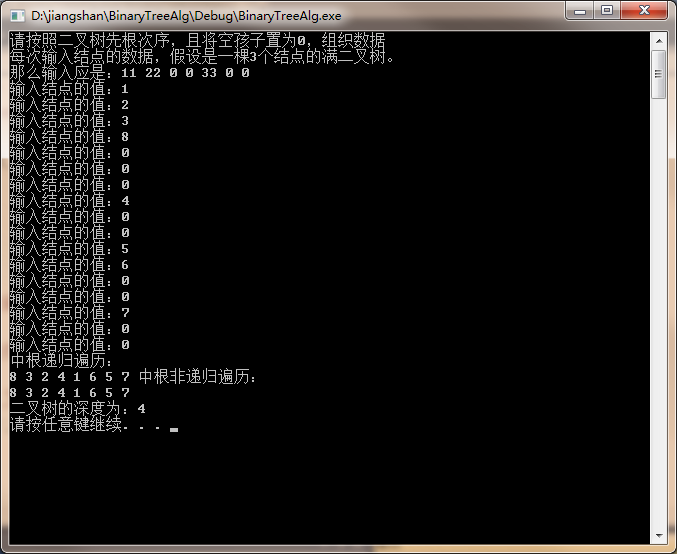• ## 二叉树建立和遍历

千次阅读 2014-07-24 15:47:04
二叉树建立和遍历
二叉树创建遍历规则:
1.先序:根-左-右
2.中序:左-根-右
3.后序:左-右-根

二叉树定义和辅助函数如下：

struct node {
int data;
struct node* left;
struct node* right;
};

void visit(int data)
{
printf("%d ", data);
}

int indata()
{
int data;
scanf("%d",&data);
return data;
}

先序创建二叉树:

struct node* CreateBiTree()//先序建立一个二叉树
{
char x; //x为根节点
struct node* t;
x=indata();
if (x==' ') /* 判断当前子树是否创建完成*/
return NULL;
else
{
t=(struct node*)malloc(sizeof(struct node));
t->data=x;
t->left=CreateBiTree();
t->right=CreateBiTree();
}
return t;
}  
先序遍历二叉树：

void preOrder(struct node* root)
{
if (root == NULL)
return;
visit(root->data);
preOrder(root->left);
preOrder(root->right);
}


中序创建二叉树:

struct node* CreateBiTree()//先序建立一个二叉树
{
char x; //x为根节点
struct node* t;
x=indata();
if (x==' ') /* 判断当前子树是否创建完成*/
return NULL;
else
{
t=(struct node*)malloc(sizeof(struct node));
t->left=CreateBiTree();
t->data=x;
t->right=CreateBiTree();
}
return t;
}  
中序遍历二叉树:

void inOrder(struct node* root)
{
if (root == NULL)
return;
inOrder(root->left);
visit(root->data);
inOrder(root->right);
}  

后序创建二叉树

struct node* CreateBiTree()//先序建立一个二叉树
{
char x; //x为根节点
struct node* t;
x=indata();
if (x==' ') /* 判断当前子树是否创建完成*/
return NULL;
else
{
t=(struct node*)malloc(sizeof(struct node));
t->left=CreateBiTree();
t->right=CreateBiTree();
t->data=x;
}
return t;
}

后序遍历二叉树

void inOrder(struct node* root)
{
if (root == NULL)
return;
inOrder(root->left);
inOrder(root->right);
visit(root->data);
}  

转载请注明作者：小刘


展开全文创建
• 大型二叉树建立遍历系统\大型二叉树建立遍历系统.
• 这篇换了一种新的建立方法，用先根遍历递归的思路建立二叉树，用递归的方法计算深度，用中根递归非递归方法遍历整个二叉树。 BinaryTree.h //二叉树建立和遍历 #ifndef BINARYTREE_H_ #define BINARYTREE_H_ #...
上篇咱们说到二叉树的一种建立方法及三种遍历方法的递归非递归算法。这篇换了一种新的建立方法，用先根遍历递归的思路建立二叉树，用递归的方法计算深度，用中根递归和非递归方法遍历整个二叉树。
BinaryTree.h

//二叉树的建立和遍历
#ifndef BINARYTREE_H_
#define BINARYTREE_H_
#include <iostream>
typedef int T;
struct Node
{
T data;
Node *lch;
Node *rch;
};
class BinaryTree
{
private:
Node *root;
Node *create_bt();
void inorder(Node *p);
int predepth(Node *t);
void destroy(Node *p);
int max(int x, int y);
public:
BinaryTree()
{
root=NULL;
}
~BinaryTree()
{
destroy(root);
}
void create();
void inorder()
{
inorder(root);
}
void inorderz();//中根非递归遍历
int predepth(){return predepth(root);}//求二叉树的深度递归函数
};

#endifBinaryTree.cpp

#include "BinaryTree.h"
#include <iostream>
#include <stack>

using namespace std;

void BinaryTree::create()
{
cout<<"请按照二叉树先根次序，且将空孩子置为0，组织数据"<<endl;
cout<<"每次输入结点的数据，假设是一棵3个结点的满二叉树。"<<endl;
cout<<"那么输入应是：11 22 0 0 33 0 0"<<endl;
root=create_bt();
}
Node *BinaryTree::create_bt()
{
Node *t;
int e;
cout<<"输入结点的值：";
cin>>e;
if(e==0)
t=NULL;
else
{
t=new Node;
t->data=e;
t->lch=create_bt();
t->rch=create_bt();
}
return t;
}
void BinaryTree::inorderz()//中根非递归遍历
{
Node *p=root;
Node *q;
stack<Node *> S;
do
{
while(p!=NULL)
{
S.push(p);
p=p->lch;
}
p=S.top();
S.pop();
cout<<p->data<<" ";
p=p->rch;
}while(!(S.empty() && p==NULL));
}
void BinaryTree::inorder(Node *p)
{
if(p!=NULL)
{
inorder(p->lch);
cout<<p->data<<" ";
inorder(p->rch);
}
}
int BinaryTree::predepth(Node *t)
{
if(t!=NULL)
{
return 1+max(predepth(t->lch),predepth(t->rch));
}
else
return 0;
}
int BinaryTree::max(int x, int y)
{
return (x>=y?x:y);
}
//用递归法删除二叉树的所有结点
void BinaryTree::destroy(Node *p)
{
if(p!=NULL)
{
destroy(p->lch);
destroy(p->rch);
}
delete p;
}
main.cpp

#include <iostream>
#include "BinaryTree.h"

using namespace std;

int main()
{
BinaryTree BT;
BT.create();
cout<<"中根递归遍历："<<endl;
BT.inorder();
cout<<"中根非递归遍历："<<endl;
BT.inorderz();
cout<<endl<<"二叉树的深度为："<<BT.predepth()<<endl;
system("pause");
return 0;
}结果为：展开全文• 在学习数据结构的过程中，二叉树是一个绕不过去的问题，关于二叉树我们不仅要了解二叉树如何建立，也要了解二叉树如何进行遍历，在一些acm或者某些学校的考研复试机试中可能会涉及到二叉树。 我们在解题或者实际...
在学习数据结构的过程中，二叉树是一个绕不过去的问题，关于二叉树我们不仅要了解二叉树如何建立，也要了解二叉树如何进行遍历，在一些acm或者某些学校的考研复试机试中可能会涉及到二叉树。
我们在解题或者实际应用过程中，首先会按照先序序列去建立二叉树，其过程在各大数据结构的书籍中不尽相同，应该是这样的。
typedef struct BTNode{
char data;
struct BTNode *lchild.*rchild;
}BTNode,BiTree;

void CreateBiTree(BiTree &T) {
scanf(“%c”,&ch);
if (ch==‘# ’) T = NULL;
else {
T = (BiTNode *)malloc(sizeof(BiTNode))；
T->data = ch;              // 生成根结点
CreateBiTree(T->lchild);   // 构造左子树
CreateBiTree(T->rchild);   // 构造右子树
}
}

这段代码对很多初学者来说一定是耳熟能详的，但是这里面涉及到一个很重要的问题，就是c语言中没有引用，因此'&'的使用编译器不会通过。
于是我们进而会选择不使用&符号，学习过c语言的应该都知道，指针其实就是一个地址，修改指针的内容就相当于修改了指针指向的地址的数据，我们可以将这个函数更改为一个返回值是根节点指针的函数，看看是否可以成功呢？
BiTree CreateBiTree(BiTree T) {
scanf(“%c”,&ch);
if (ch==‘# ’) T = NULL;
else {
T = (BiTNode *)malloc(sizeof(BiTNode))；
T->data = ch;              // 生成根结点
CreateBiTree(T->lchild);   // 构造左子树
CreateBiTree(T->rchild);   // 构造右子树
}
return T;
}
经过尝试，仍旧还是无法解决这个问题，二叉树仍然无法建立起来。
后来经过查找文献和资料，发现如下的方法可以成功构造二叉树：
void createBiTree(PTree *p)//建立二叉树
{
char ch;
scanf("%c", &ch);
getchar();
if(ch == '#')
*p = NULL;
else
{
*p = (PTree)malloc(sizeof(Tree));
(*p) -> ch = ch;
createBiTree(&(*p) -> lchild);
createBiTree(&(*p) -> rchild);
}

}
通过这种方法就可以完成二叉树的建立。继而去调用其它的遍历函数，即可得到期望的结果。
展开全文c
• 数据结构（对C语言的描述）有关二叉树建立遍历（算法源代码） 包括其中序、后序、先序遍历二叉树的两种建立方法
• C实现二叉树建立及先中后序的遍历，控制台程序，在各版本vs上均可运行
• 数据结构试验3二叉树建立遍历等操作代码及运行结果。 实验内容： 采用二叉链表存储，实现二叉树的创建、遍历（递归）、赫夫曼编码译码等典型操作。 1. 编程实现如下功能： （1）假设二叉树的结点值是字符型，...
• 二叉树建立以及遍历 利用递归的方式建立二叉树 并以中序遍历的方式读取数据，根据遍历二叉树的三种方式，只需要在递归时修改访问结点的元素即可。 #include <stdio.h> #include <stdlib.h> ...
二叉树的建立以及遍历
利用递归的方式建立二叉树 并以中序遍历的方式读取数据，根据遍历二叉树的三种方式，只需要在递归时修改访问结点的元素即可。
#include <stdio.h>
#include <stdlib.h>
typedef struct Binode{
char data;
struct Binode *Rchild ,*Lchild;
}BiTnode,*BiTree;
void createBTree( BiTree *T)
{
char c ;
scanf("%c",&c);
if(' '==c){
*T=NULL;
}
else{
*T=(BiTnode*)malloc(sizeof(BiTnode));
(*T)->data=c;
createBTree(&(*T)->Lchild);
createBTree(&(*T)->Rchild);
}
}
void visit(char c ,int level){
printf("%c   %d\n",c,level);
}
void preOrderTraverse(BiTree T, int level)
{
if(T)
{
preOrderTraverse(T->Lchild,level+1);
visit(T->data,level);
preOrderTraverse(T->Rchild,level+1);
}
}
int main()
{
BiTree t ;
t=NULL;
printf("创建二叉树，以空格代表子结点为空:\n");
createBTree(&t);
preOrderTraverse(t,1);
return 0;
}


注意：在此建立二叉树使用的是指针的指针，如果用的时是指针的话，作为形参传递给函数，当函数返回后，函数出栈，也就不能 获得结点信息，而使用二重指针 头节点本身为指针，在函数递归的过程中需要修改本身的值，就需要使用二重指针，这类似于利用函数交换值得思想。

展开全文• 建立一颗带头结点的二叉树 遍历 访问函数 主代码 定义结点数据类型 typedef struct bitnode { char data; struct bitnode *Lchild,*Rchild; } BiTNode,*BiTree; 建立一颗带头结点的二叉树 BiTree ...数据结构
• 建立二叉树，实现二叉树的先序、中序、后序的递归遍历算法，输出遍历结果。 实现二叉树的先序、中序、后序层次遍历的非递归算法，输出遍历结果。
• 前两天说过二叉树的前序遍历、中序遍历、后续遍历，把剩下的也都说了吧，二叉树遍历系列四，层次遍历。题目链接：二叉树的层次遍历 - 力扣（LeetCode）​leetcode-cn.com题目描述：给定一个二叉树，返回其按层次遍历...
• 线索二叉树建立，前中后线索的建立，前中后序递归非递归遍历，广义表形式输出，层序遍历
• 链表实现二叉树创建和遍历，三种遍历方式实现，二叉树
• C语言_二叉树建立遍历、交换子树代码、是可执行的源代码，欢迎大家下载C语言
• 二叉树建立，手工输入树，可以遍历遍历方法有：前序，中序后序 。
• 线索二叉树建立遍历 线索二叉树概念 二叉树通过二叉链表作为存储结构时，只能得到当前结点的左右孩子信息，而无法得到结点在任一序列的前驱后继，如果想要保存遍历中的信息,需要在每个结点上添加两个指针域...
• 本文首发于公众号「五分钟学算法」，是图解 LeetCode 系列文章之一。 个人网站：https://www.cxyxiaowu.com题目来源于 LeetCode ... （即按从叶子节点所在层到根节点所在的层，逐层从左向右遍历）例如： 给定二叉树 ...
• 题目描述 二叉树的前序、中序、后序遍历的定义： 前序遍历：对任一子树，先访问跟... 给定一棵二叉树的前序遍历和中序遍历，求其后序遍历（提示：给定 前序遍历与中序遍历能够唯一确定后序遍历）。 输入 两个
• 建立二叉树 先序遍历 中序遍历 后序遍历 层序遍历 二叉树 二叉树是树形结构的一种特殊形式，它的特点是每个结点至多只有两颗子树（即二叉树中不存在度大于2的结点），并且，二叉树的子树有左右之分，其次...
• c语言几种建立遍历方式，仅供参考，在vc++6.0环境下可以运行！
• 二叉树建立及非递归遍历，包含先序、中序、后序三种
• 最近在学习二叉树，遇到了这样一题，在这里给大家提供一种方法，可能不是最好的，仅供大家参考相互交流学习。 已知一个二叉树前序遍历、中序遍历的结果，请确定该二叉树并输出其后序遍历的结果。 例如: 先序遍历...Python...